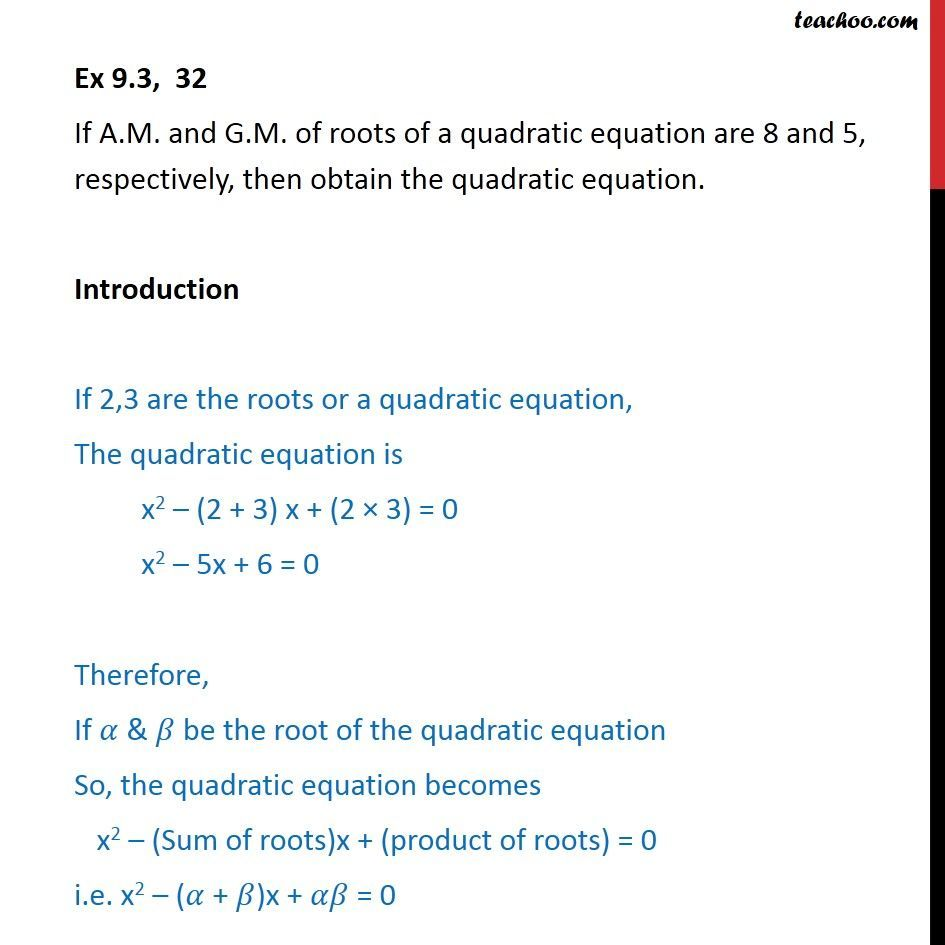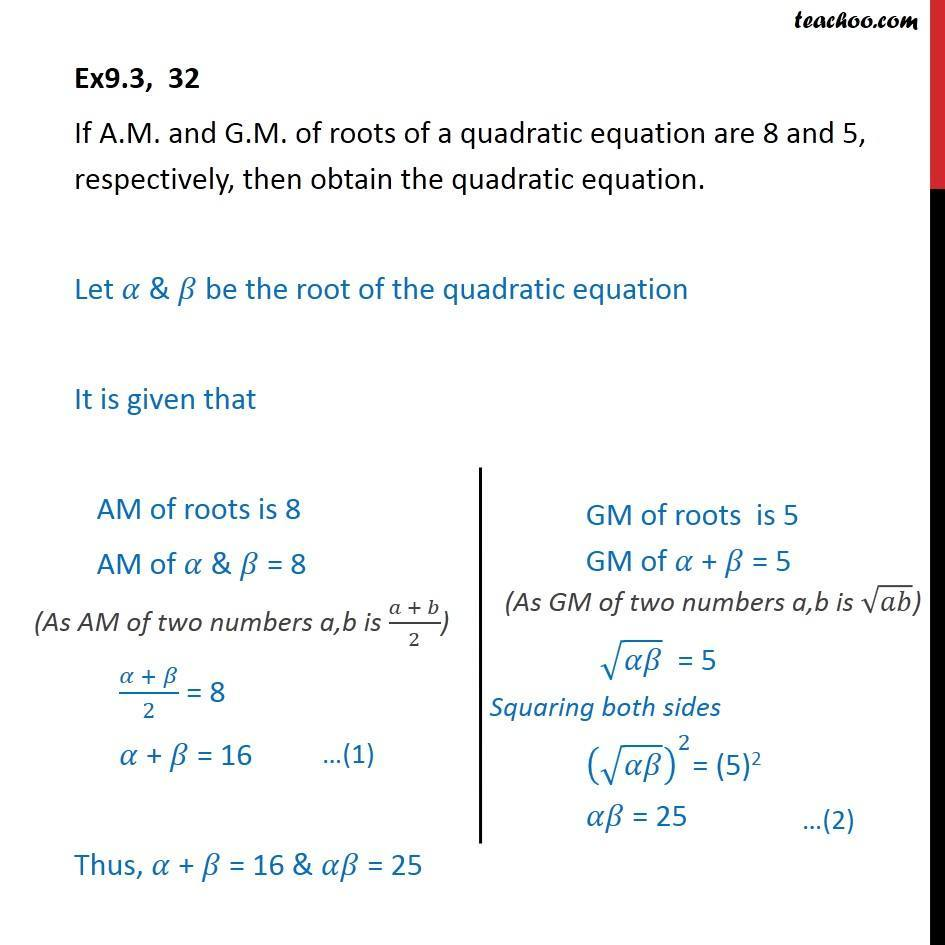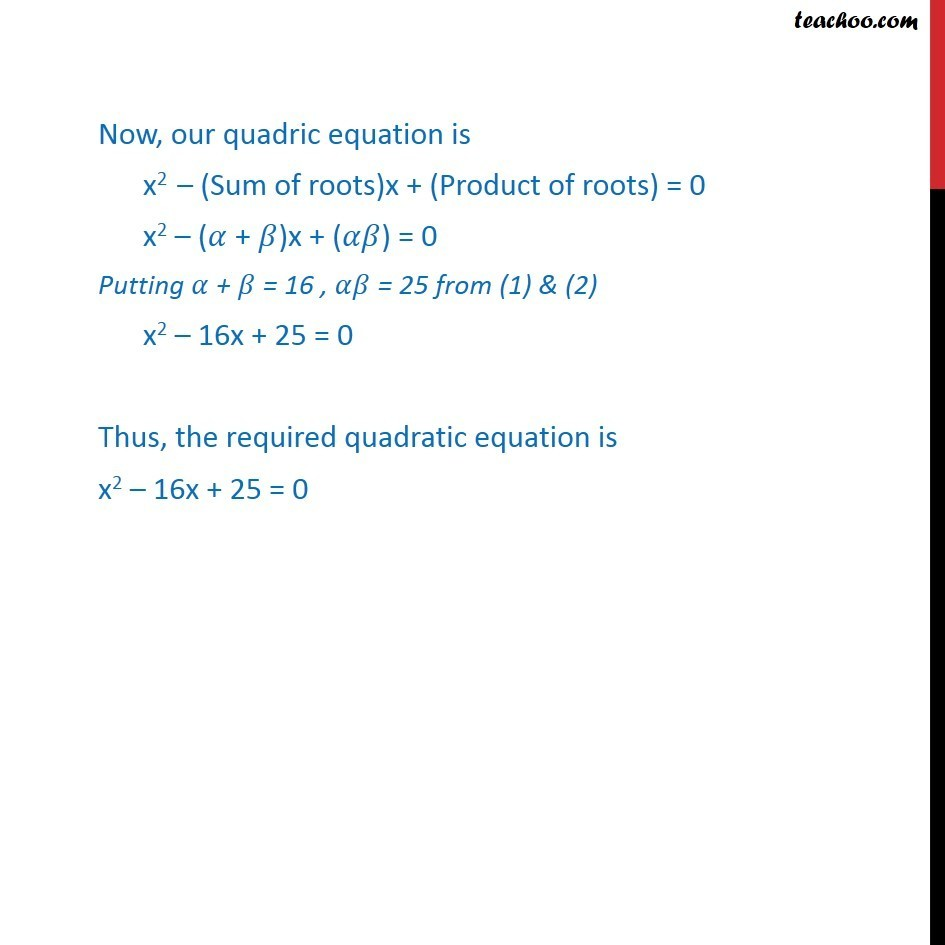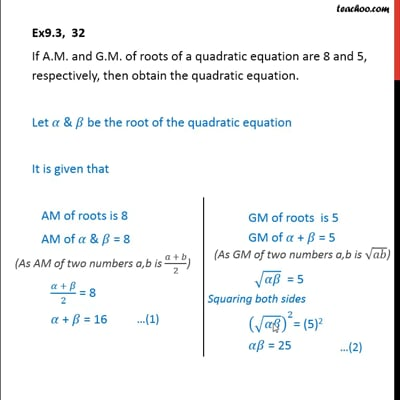Ex 9.3

Chapter 9 Class 11 Sequences and Series
Serial order wiseThis video is only available for Teachoo black users

Solve all your doubts with Teachoo Black (new monthly pack available now!)

### Transcript

Ex 9.3, 32 If A.M. and G.M. of roots of a quadratic equation are 8 and 5, respectively, then obtain the quadratic equation. Introduction If 2,3 are the roots or a quadratic equation, The quadratic equation is x2 – (2 + 3) x + (2 × 3) = 0 x2 – 5x + 6 = 0 Therefore, If 𝛼 & 𝛽 be the root of the quadratic equation So, the quadratic equation becomes x2 – (Sum of roots)x + (product of roots) = 0 i.e. x2 – (𝛼 + 𝛽)x + 𝛼𝛽 = 0 Ex9.3, 32 If A.M. and G.M. of roots of a quadratic equation are 8 and 5, respectively, then obtain the quadratic equation. Let 𝛼 & 𝛽 be the root of the quadratic equation It is given that AM of roots is 8 AM of 𝛼 & 𝛽 = 8 (𝛼 + 𝛽)/2 = 8 𝛼 + 𝛽 = 16 Thus, 𝛼 + 𝛽 = 16 & 𝛼𝛽 = 25 Now, our quadric equation is x2 – (Sum of roots)x + (Product of roots) = 0 x2 – (𝛼 + 𝛽)x + (𝛼𝛽) = 0 Putting 𝛼 + 𝛽 = 16 , 𝛼𝛽 = 25 from (1) & (2) x2 – 16x + 25 = 0 Thus, the required quadratic equation is x2 – 16x + 25 = 0